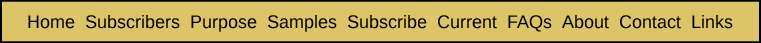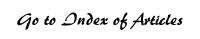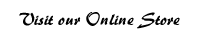The Markov Chain ELS Extension Model Determining How Many Long ELSs Would Be Expected to Be Found in a Non-Encoded Text Appendix Seven from Bible Code Bombshell After a Hebrew expert examines the string of letters resulting from taking every j-th letter before and after the initial ELS with a skip of j letters, one of two results will occur in each instance that there is an opportunity to find an extension of the initial ELS. If no extension is found, this will be denoted by [Nothing] and if one is found, by [Extension]. So the Hebrew expert starts with: [preceding letters] [Initial ELS] [succeeding letters]. Four possible things can happen: [Nothing] [Initial ELS] [Nothing] [Nothing] [Initial ELS] [Extension] [Extension] [Initial ELS] [Nothing] [Extension] [Initial ELS] [Extension]. To shorten things these outcomes are denoted by: NIN NIE EIN EIE. Once an N occurs, the search process is over on that side of the initial ELS. But if an extension is found, there is an opportunity to find yet another extension. So if NIN happens, the search is over. But if NIE happens, two things could result from the additional search (NIEN and NIEE). If NIEN occurs, the process is over. But if NIEE occurs, two things could result from the additional search (NIEEN and NIEEE). And so forth. By calculating the probability that each of these events could occur by chance, and organizing the results in a certain way, it is possible to simplify all this down to a straightforward formula. This is done in the last section of this appendix. The formula for the expected number of ELSs with k extensions to emerge from an examination of an initial group of n ELSs found in a text is given in Figure App7A. Figure App7A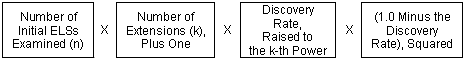As an example of usage of the formula in Figure App7A, suppose we reviewed 100 initial ELSs for extensions and that the extension discovery rate was 20%. Then, we should expect that we would end up with 25.6 final ELSs consisting of exactly one extension: 100 x [1 + 1] x [20%]^1 x [80%]^2, or 100 x 2 x 20% x 64% = 25.6. And we should expect that we would end up with 7.68 final ELSs consisting of exactly two extensions: 100 x [ 2+ 1] x [20%]^2 x [80%]^2, or 100 x 3 x 4% x 64% = 7.68. And so forth. The purpose of all this is to define a benchmark of how many long ELSs you would expect to find if you searched a presumably non-encoded Hebrew text. Having that benchmark, a standard statistical procedure, called the chi-square test, can be applied to estimate the probability of chance occurrence of the actual results differing from the expected benchmark. Technical Derivation of the Markov Chain ELS Extension Model This section of the appendix provides a derivation of the formula shown in Figure App7A. Figure App7B presents the complete range of outcomes from the examination process up through the location of three extensions to an initial ELS. From this chart, the formula for determining the expected number of ELSs with a given number of extensions is evident Each outcome is represented first by a combination of the letters I, E and N, indicating the order in which these events appeared in that letter string. In the middle section of the table is a row of formulae for the expected number of ELSs for each type of outcome in that column. In each formula, n is the total number of initial ELSs, d is the probability of finding a grammatically correct Hebrew extension of the preceding ELS, and (1–d) is the probability of failing to find an extension. It was assumed that d is independent of the number of extensions that have already been discovered � even though consideration of the different factors affecting d as the number of extensions increases suggests that d most likely declines as the ELS becomes longer. Figure App7A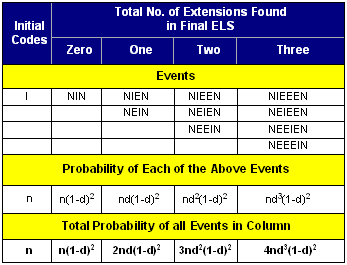The beginning of the search process is represented by the leftmost column of the table, where there are n instances where I, an initial ELS, appears. Each column represents the range of outcomes that would result in the indicated number of final extensions. The only way that zero extensions will be found is the situation where no extension is found either before or after the initial ELS (denoted by NIN). The probability of this occurring for any given initial ELS is (1–d)2. So the expected number of final ELSs that have no extension is n(1–d)2. There are two ways that a final ELS can have one and only one extension: NIEN and NEIN. The expected number of final ELSs that are of the NIEN type is nd(1–d)2, as is the case for the NEIN type. So the total expected number of final ELSs with exactly one extension is 2nd(1–d)2, as shown at the bottom of the "One" column. There are three ways that a final ELS can have exactly two extensions: NIEEN, NEIEN and NEEIN. For any given column (representing k extensions), I can appear in the 1st, 2nd . . . or (k+1)st position after the first N. Thus the total expected number of final ELSs with exactly k extensions will be (1) (k+1)ndk(1–d)2. The above model is an example of a Markov chain, since the outcome of any trial depends at most on the outcome of the immediately preceding trial and not upon any other previous outcome, and the probability of each state is clearly defined. When the state N occurs, it is an "absorbing state." Formula (1) for the expected number of ELSs with k extensions to emerge from an examination of an initial group of n ELSs found in a text has already appeared Appendix Five and earlier in this appendix. It is repeated here.Verifying the Formula As a check on formula (1), it should be possible to show that the sum of (1) for k ranging from 0 to infinity is n, the total number of initial ELSs. That derivation is as follows: Since n and (1–d)2 appear in each term, we can factor them out of an expression for the total number of final ELSs, to get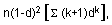where k ranges from 0 to infinity. If we multiply each term of this power series by d in both the numerator and denominator, we obtain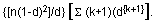If we shift the value of (k+1) by one for each term, so that the series is summed from k = 1 to infinity, rather than from k = 0 to infinity, it becomes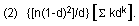According to formula 40 on page 8 of Summation of Series, collected by L.B.W. Jolley: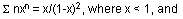the series is summed from n=1 to infinity. If we substitute k for n and d for x in this formula, we have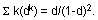By substituting the expression on the right for the power series expression in (2) above, we getEnjoy finding your own Bible codes. Bible code search software is available in our online store. Subscribe Free! By signing up to be a member of The Isaac Newton Bible Research Society, you will have access to more than fifteen years of research by our team of Bible code researchers. Sign up to be a member today.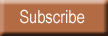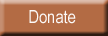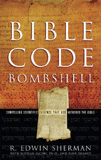Bombshell examines two massive, recently discovered clusters of codes in the Hebrew Old Testament. To read more about Bombshell, click here, or click below to order from Amazon today!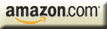| HOME | SUBSCRIBERS ONLY | OUR PURPOSE | FREE SAMPLES | CURRENT | | FAQs | ABOUT BIBLE CODES | SUBSCRIBE | CONTACT | Copyright © 2016 BibleCodeDigest.com